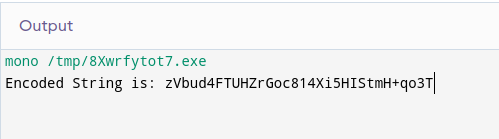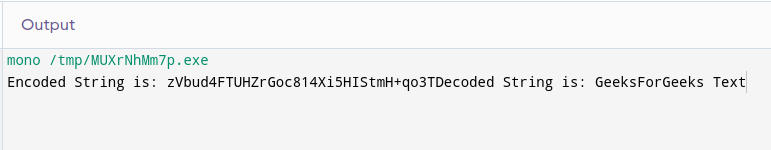Open in App
Not now

# Encrypt and Decrypt Using Rijndael Key in C#

• Difficulty Level : Hard
• Last Updated : 21 Nov, 2022

To keep data secure and protected it is necessary to keep the data encrypted. As we know that in C# and in other languages too there are many ways for encrypting data. The Data Encryption Standard method used for encryption was not promising good security that led to the invention of a highly secure tool called Rijndael Key by Vincent Rijmen and Joan Daemon. In this article, we will learn about the Rijndael key and perform step-by-step Encryption and Decryption of certain data by using Rijndael Key in C#.

## Block Cipher:

A block cipher is a method of encrypting data in blocks for producing a cipher text using a cryptographic key and an algorithm. block ciphers are more secure and reliable than Standard Data Encryption (DES).

## Rijndael Key:

Rijndael is based on the block cipher method which uses a symmetric key encryption technique. It works with the help of invertible and discrete layers

• Linear Mix Transform
• Non – Linear Transform

As for C#, the Rijndael key supports key lengths of 128, 192, and 256 bits and also supports blocks of 128 (by default), 192, and 256 bits. Rijndael key is very much similar to AES(Advance Encryption Standard).

## Implementation of Encryption of a String:

Step 1: The first step would be to create a C# file in the IDE of your choice or you can just use the GeeksForGeeks IDE. Name the Class “GFGEncryption” to keep things simple and aligned with the tutorial.

Step 2:Now make a new method named encodeString( ) which takes no parameters and returns a string.

Step 3: Now inside the blocks of encodeString method, we will write the actual code for encoding our string. we will use the string “GeeksForGeeks Text”. We will encode this string. Inside the method, we have to create multiple variables.

```Note :
The public and private key both must have at least 8 characters.
Don't forget to add import statements.```

Example:

## C#

 `using` `System.IO;``using` `System.Security.Cryptography;``using` `System.Text;` `public` `class` `GFGEncryption{` `    ``static` `public` `void` `Main (){` `    ``}``  ` `    ``public` `static` `string` `encodeString (){``        ``string` `data = ``"GeeksForGeeks Text"``;``        ``string` `answer = ``""``;``        ``string` `publicKey = ``"GEEK1234"``;``        ``string` `privateKey = ``"PKEY4321"``;``        ``byte``[] privatekeyBytes = Encoding.UTF8.GetBytes(privateKey);``        ``byte``[] publicKeyBytes = Encoding.UTF8.GetBytes(publicKey);``        ``byte``[] inputByteArray= System.Text.Encoding.UTF8.GetBytes(data);``        ``return` `answer;``    ``}``}`

Step 4: Once we have created all the required variables we can now perform the actual encoding operation by using the class called “DESCryptoServiceProvider”. Now inside the block of this class, we will create two new objects of the type

• MemoryStream
• CryptoStream

We will use the Write method from CryptoStream class and pass the input byte array and its length into it resulting in an encoded array. Your code must look as below.

Example:

## C#

 `using` `System.IO;``using` `System.Security.Cryptography;``using` `System.Text;``using` `System;` `public` `class` `GFGEncryption{` `    ``static` `public` `void` `Main (){` `    ``}``  ` `    ``public` `static` `string` `encodeString (){``        ``string` `data = ``"GeeksForGeeks Text"``;``        ``string` `answer = ``""``;``        ``string` `publicKey = ``"GEEK1234"``;``        ``string` `privateKey = ``"PKEY4321"``;``        ``byte``[] privateKeyBytes ={};``        ``privateKeyBytes = Encoding.UTF8.GetBytes(privateKey);``        ``byte``[] publicKeyBytes = {};``        ``publicKeyBytes = Encoding.UTF8.GetBytes(publicKey);``        ``byte``[] inputByteArray= System.Text.Encoding.UTF8.GetBytes(data);``        ``using` `(DESCryptoServiceProvider provider = ``new` `DESCryptoServiceProvider())``                ``{``                    ``var` `memoryStream = ``new` `MemoryStream();``                    ``var` `cryptoStream = ``new` `CryptoStream(memoryStream,``                    ``provider.CreateEncryptor(publicKeyBytes, privateKeyBytes),``                     ``CryptoStreamMode.Write);``                    ``cryptoStream.Write(inputByteArray, 0, inputByteArray.Length);``                    ``cryptoStream.FlushFinalBlock();``                    ``answer = Convert.ToBase64String(memoryStream.ToArray());``                ``}``        ``return` `answer;``    ``}``}`

Step 5: Finally, we have successfully implemented the encodeString( ) method and we are going to use it in our main class. You should use the method as shown below

Example:

## C#

 `using` `System.IO;``using` `System.Security.Cryptography;``using` `System.Text;``using` `System;` `public` `class` `GFGEncryption{` `    ``static` `public` `void` `Main (){``      ``string` `encryptedString = encodeString();``            ``Console.Write(``"Encoded String is: "` `+encryptedString);``    ``}``  ` `    ``public` `static` `string` `encodeString(){``        ``string` `data = ``"GeeksForGeeks Text"``;``        ``string` `answer = ``""``;``        ``string` `publicKey = ``"GEEK1234"``;``        ``string` `privateKey = ``"PKEY4321"``;``        ``byte``[] privateKeyBytes ={};``        ``privateKeyBytes = Encoding.UTF8.GetBytes(privateKey);``        ``byte``[] publicKeyBytes = {};``        ``publicKeyBytes = Encoding.UTF8.GetBytes(publicKey);``        ``byte``[] inputByteArray= System.Text.Encoding.UTF8.GetBytes(data);``        ``using` `(DESCryptoServiceProvider provider = ``new` `DESCryptoServiceProvider())``                ``{``                    ``var` `memoryStream = ``new` `MemoryStream();``                    ``var` `cryptoStream = ``new` `CryptoStream(memoryStream,``                    ``provider.CreateEncryptor(publicKeyBytes, privateKeyBytes),``                    ``CryptoStreamMode.Write);``                    ``cryptoStream.Write(inputByteArray, 0, inputByteArray.Length);``                    ``cryptoStream.FlushFinalBlock();``                    ``answer = Convert.ToBase64String(memoryStream.ToArray());``                ``}``        ``return` `answer;``    ``}``}`

Output :## Decryption of a String:

Step 1: Similarly, as we created an encoded string method, we will create a decodeString( ) method which decodes the given encrypted string and returns its true value. create the decodeString( ) method just like shown below.

Example:

## C#

 `using` `System.IO;``using` `System.Security.Cryptography;``using` `System.Text;``using` `System;` `public` `class` `GFGEncryption{` `    ``static` `public` `void` `Main (){``      ``string` `encryptedString = encodeString();``            ``Console.Write(``"Encoded String is: "` `+encryptedString);``    ``}``  ` `    ``public` `static` `string` `encodeString(){``        ``string` `data = ``"GeeksForGeeks Text"``;``        ``string` `answer = ``""``;``        ``string` `publicKey = ``"GEEK1234"``;``        ``string` `privateKey = ``"PKEY4321"``;``        ``byte``[] privateKeyBytes ={};``        ``privateKeyBytes = Encoding.UTF8.GetBytes(privateKey);``        ``byte``[] publicKeyBytes = {};``        ``publicKeyBytes = Encoding.UTF8.GetBytes(publicKey);``        ``byte``[] inputByteArray= System.Text.Encoding.UTF8.GetBytes(data);``        ``using` `(DESCryptoServiceProvider provider = ``new` `DESCryptoServiceProvider())``                ``{``                    ``var` `memoryStream = ``new` `MemoryStream();``                    ``var` `cryptoStream = ``new` `CryptoStream(memoryStream,``                     ``provider.CreateEncryptor(publicKeyBytes, privateKeyBytes),``                     ``CryptoStreamMode.Write);``                    ``cryptoStream.Write(inputByteArray, 0, inputByteArray.Length);``                    ``cryptoStream.FlushFinalBlock();``                    ``answer = Convert.ToBase64String(memoryStream.ToArray());``                ``}``        ``return` `answer;``    ``}``  ` `    ``public` `static` `string` `decodeString(String str) {``      ``string` `answer = ``""``;``      ``return` `answer;``    ``}``}`

Step 2: Now inside the decode method add all the variables just like we created in the encodeString( ) method. This time instead of creating a separate variable “data” we will directly use the data variable which is coming from methods parameters. Do it as demonstrated.

Example:

## C#

 `using` `System.IO;``using` `System.Security.Cryptography;``using` `System.Text;``using` `System;` `public` `class` `GFGEncryption{` `    ``static` `public` `void` `Main (){``      ``string` `encryptedString = encodeString();``            ``Console.Write(``"Encoded String is: "` `+encryptedString);``    ``}``  ` `    ``public` `static` `string` `encodeString(){``        ``string` `data = ``"GeeksForGeeks Text"``;``        ``string` `answer = ``""``;``        ``string` `publicKey = ``"GEEK1234"``;``        ``string` `privateKey = ``"PKEY4321"``;``        ``byte``[] privateKeyBytes ={};``        ``privateKeyBytes = Encoding.UTF8.GetBytes(privateKey);``        ``byte``[] publicKeyBytes = {};``        ``publicKeyBytes = Encoding.UTF8.GetBytes(publicKey);``        ``byte``[] inputByteArray= System.Text.Encoding.UTF8.GetBytes(data);``        ``using` `(DESCryptoServiceProvider provider = ``new` `DESCryptoServiceProvider())``                ``{``                    ``var` `memoryStream = ``new` `MemoryStream();``                    ``var` `cryptoStream = ``new` `CryptoStream(memoryStream,``                    ``provider.CreateEncryptor(publicKeyBytes, privateKeyBytes),``                    ``CryptoStreamMode.Write);``                    ``cryptoStream.Write(inputByteArray, 0, inputByteArray.Length);``                    ``cryptoStream.FlushFinalBlock();``                    ``answer = Convert.ToBase64String(memoryStream.ToArray());``                ``}``        ``return` `answer;``    ``}``  ` `    ``public` `static` `string` `decodeString(String data) {``      ``string` `answer = ``""``;``      ``string` `publicKey = ``"GEEK1234"``;``        ``string` `privateKey = ``"PKEY4321"``;``        ``byte``[] privateKeyBytes ={};``        ``privateKeyBytes = Encoding.UTF8.GetBytes(privateKey);``        ``byte``[] publicKeyBytes = {};``        ``publicKeyBytes = Encoding.UTF8.GetBytes(publicKey);``        ``byte``[] inputByteArray= ``new` `byte``[data.Replace(``" "``, ``"+"``).Length];``        ``using` `(DESCryptoServiceProvider provider = ``new` `DESCryptoServiceProvider())``                ``{``                    ``var` `memoryStream = ``new` `MemoryStream();``                    ``var` `cryptoStream = ``new` `CryptoStream(memoryStream,``                    ``provider.CreateEncryptor(publicKeyBytes, privateKeyBytes),``                    ``CryptoStreamMode.Write);``                    ``cryptoStream.Write(inputByteArray, 0, inputByteArray.Length);``                    ``cryptoStream.FlushFinalBlock();``                    ``answer = Encoding.UTF8.GetString(memoryStream.ToArray());``                ``}``      ``return` `answer;``    ``}``}`

Step 3: As we have successfully implemented the method for decrypting the encoded string we will use the method in the main function and see if the decoded value is true or not. Remember we used the string “GeeksForGeeks Text”. If we this string as a result we can conclude that we have successfully performed encryption and decryption in C#.

Example:

## C#

 `using` `System.IO;``using` `System.Security.Cryptography;``using` `System.Text;``using` `System;` `public` `class` `GFGEncryption{` `    ``static` `public` `void` `Main (){``      ``string` `encryptedString = encodeString();``      ``string` `decryptedString = decodeString(encryptedString);``      ``Console.Write(``"Encoded String is: "` `+encryptedString);``      ``Console.Write(``"\nDecoded String is: "` `+decryptedString);``    ``}``  ` `    ``public` `static` `string` `encodeString(){``        ``string` `data = ``"GeeksForGeeks Text"``;``        ``string` `answer = ``""``;``        ``string` `publicKey = ``"GEEK1234"``;``        ``string` `privateKey = ``"PKEY4321"``;``        ``byte``[] privateKeyBytes ={};``        ``privateKeyBytes = Encoding.UTF8.GetBytes(privateKey);``        ``byte``[] publicKeyBytes = {};``        ``publicKeyBytes = Encoding.UTF8.GetBytes(publicKey);``        ``byte``[] inputByteArray= System.Text.Encoding.UTF8.GetBytes(data);``        ``using` `(DESCryptoServiceProvider provider = ``new` `DESCryptoServiceProvider())``                ``{``                    ``var` `memoryStream = ``new` `MemoryStream();``                    ``var` `cryptoStream = ``new` `CryptoStream(memoryStream,``                    ``provider.CreateEncryptor(publicKeyBytes, privateKeyBytes),``                    ``CryptoStreamMode.Write);``                    ``cryptoStream.Write(inputByteArray, 0, inputByteArray.Length);``                    ``cryptoStream.FlushFinalBlock();``                    ``answer = Convert.ToBase64String(memoryStream.ToArray());``                ``}``        ``return` `answer;``    ``}``  ` `    ``public` `static` `string` `decodeString(String data) {``      ``string` `answer = ``""``;``      ``string` `publicKey = ``"GEEK1234"``;``        ``string` `privateKey = ``"PKEY4321"``;``        ``byte``[] privateKeyBytes ={};``        ``privateKeyBytes = Encoding.UTF8.GetBytes(privateKey);``        ``byte``[] publicKeyBytes = {};``        ``publicKeyBytes = Encoding.UTF8.GetBytes(publicKey);``        ``byte``[] inputByteArray= ``new` `byte``[data.Replace(``" "``, ``"+"``).Length];``        ``inputByteArray = Convert.FromBase64String(data.Replace(``" "``, ``"+"``));``        ``using` `(DESCryptoServiceProvider provider = ``new` `DESCryptoServiceProvider())``                ``{``                    ``var` `memoryStream = ``new` `MemoryStream();``                    ``var` `cryptoStream = ``new` `CryptoStream(memoryStream,``                    ``provider.CreateDecryptor(publicKeyBytes, privateKeyBytes),``                    ``CryptoStreamMode.Write);``                    ``cryptoStream.Write(inputByteArray, 0, inputByteArray.Length);``                    ``cryptoStream.FlushFinalBlock();``                    ``answer = Encoding.UTF8.GetString(memoryStream.ToArray());``                ``}``      ``return` `answer;``    ``}``}`

#### Output:My Personal Notes arrow_drop_up